Electron. J. Differential Equations, Vol. 2019 (2019), No. 61, pp. 1-18.

### Decay rate of strong solutions to compressible Navier-Stokes-Poisson equations with external force Yeping Li, Nengqiu Zhang

Abstract:
In this article, we consider the three dimensional compressible Navier-Stokes-Poisson equations with the effect of external potential force. First, the stationary solution is established by solving a nonlinear elliptic system. Next, we show global well-posedness of the strong solutions for the initial value problem to the three dimensional compressible Navier-Stokes-Poisson equations when the initial data are close to the stationary solution in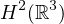. Moreover, if the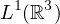-norm of initial perturbation is finite, we prove the optimal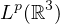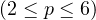decay rates for such strong solution and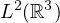decay rate of its first-order spatial derivatives via a low frequency and high frequency decomposition.

Submitted October 7, 2018. Published May 7, 2019.
Math Subject Classifications: 35M20, 35Q35, 76W05.
Key Words: Navier-Stokes-Poisson equation; stationary solution; strong solution; energy estimate; optimal decay rate.

Show me the PDF file (365 KB), TEX file for this article.Yeping Li Department of Mathematics East China University of Science and Technology Shanghai 200237, China email: yplee@ecust.edu.cn Nengqiu Zhang Department of Mathematics East China University of Science and Technology Shanghai 200237, China email: 731835397@qq.com